NCERT Class 9 Maths Quadrilaterals

# NCERT Class 9 Maths Quadrilaterals

The chapter 8 starts with an introduction to quadrilaterals by laying out a few examples followed by angle sum property of quadrilaterals. Next, the chapter   about types of quadrilaterals and properties of the parallelogram which includes a series of theorems. Finally, the chapter ends by discussing another condition for a quadrilateral to be a parallelogram followed by the midpoint theorem.

Download FREE PDF of Chapter-8 Quadrilaterals

## Chapter 8 Ex.8.1 Question 1

The angles of quadrilateral are in the ratio $$3:5:9:13$$. Find all the angles of the quadrilateral.

### Solution

What is known?

The angles of quadrilateral are in the ratio $$3:5:9:13.$$

What is unknown?

All the angles of the quadrilateral.

Reasoning:

Sum of angles in any quadrilateral is $$360$$ degree.

Steps:

Let the common ratio between the angles be $$x$$.

Therefore, the angles will be $$3x$$, $$5x$$, $$9x$$, and $$13x$$ respectively. according to the given ratio.

As the sum of all interior angles of a quadrilateral is $$360^\circ$$ ,

\begin{align} ∴\;3 x+5 x+9 x+13 x &=360^{\circ}\\30 x&=360^{\circ} \\x&=12^{\circ}\end{align}

Hence, the angles are

\begin{align}3 x&=3 \times 12=36^{\circ} \\ 5 x&=5 \times 12=60^{\circ} \\ 9 x&=9 \times 12=108^{\circ} \\ 13 x&=13 \times 12=156^{\circ}\end{align}

## Chapter 8 Ex.8.1 Question 2

If the diagonals of a parallelogram are equal, then show that it is a rectangle.

### Solution

What is known/?

The diagonals of a parallelogram are equal.

What is unknown?

How we can show that it is a rectangle.

Reasoning:

To show that given parallelogram is a rectangle, we have to prove that one of its interior angles is and this can be done by showing two triangles congruent.

Steps: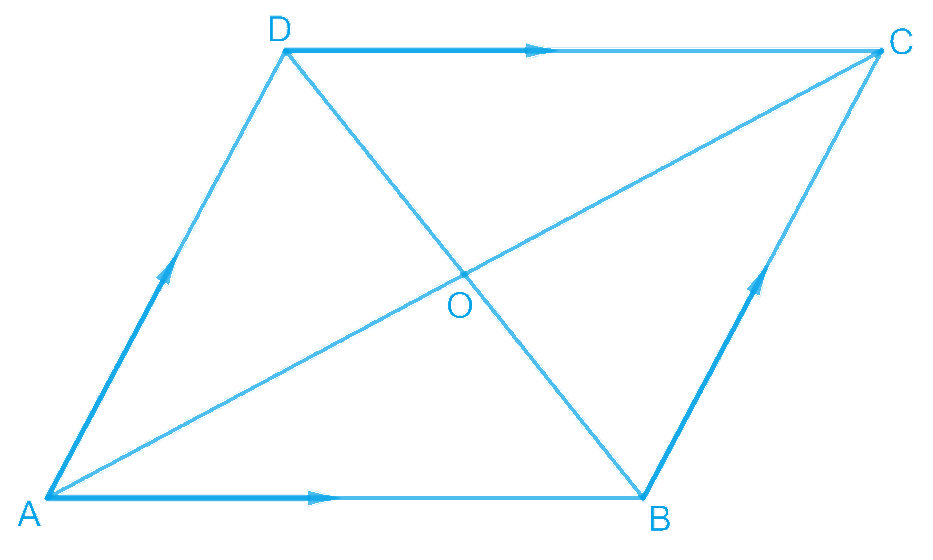Let $$ABCD$$ be a parallelogram. To show that $$ABCD$$ is a rectangle, we have to prove that one of its interior angles is \begin{align}90^{\circ}\end{align} .

In $$\Delta ABC$$ and  $$\Delta DCB$$

\begin{align} & AB=DC\, \\ & \left(\!\begin{array} & \text{In a parallelogram, } \\ \text{opposite sides are equal}\text{.} \\ \end{array}\!\right) \\ & \\ & BC=BC\,\left( \text{Common} \right) \\ & AC=DB\,\left( \text{Given} \right) \\ & \therefore \Delta ABC\,\cong \,\Delta DCB \\ & \left( By\,SSS\,\text{Congruence rule} \right) \\ & \\ & \Rightarrow \,\,\,\angle ABC=\angle DCB \\ \end{align}

It is known that the sum of the measures of angles on the same side of transversal is $$180^\circ$$.

\begin{align}\angle &{\rm{ABC}} + \angle {\rm{DCB}} = {\rm{18}}{0^0}\;\left( {{\rm{AB }}||{\rm{ CD}}} \right)\\&\Rightarrow {\rm{ }}\angle {\rm{ABC}} + \angle {\rm{ABC}} = {\rm{18}}{0^0}\\&\Rightarrow {\rm{ 2}}\angle {\rm{ABC}} = {\rm{18}}{0^0}\\& \Rightarrow {\rm{ }}\angle {\rm{ABC}} = {\rm{9}}{0^0}{\rm{ }}\end{align}

Since $$ABCD$$ is a parallelogram and one of its interior angles is \begin{align}90^{\circ},\end{align} $$ABCD$$ is a rectangle.

## Chapter 8 Ex.8.1 Question 3

Show that if the diagonals of a quadrilateral bisect each other at right angles, then it is a rhombus.

### Solution

What is known?

The diagonals of a quadrilateral bisect each other at right angles.

What is unknown?

How we can show that it is a rhombus.

Reasoning:

To show that given quadrilateral is a rhombus, we have to show it is a parallelogram and all the sides are equal.

Steps: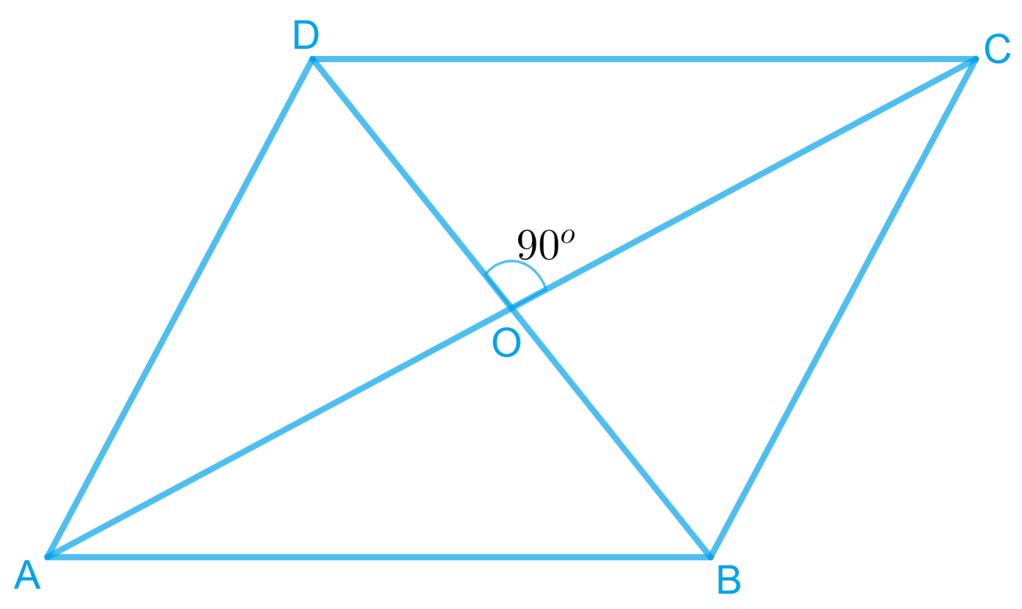Let  be a quadrilateral, whose diagonals  and  bisect each other at right angle i.e

$$\mathrm{OA}\!=\!\mathrm{OC}, \mathrm{OB}\!=\!\mathrm{OD},$$ and

$$\angle \mathrm{AOB}\!=\!\angle \mathrm{BOC}\!=\!\angle \mathrm{COD}\!=\!\angle \mathrm{AOD}\!=\!90^{\circ}$$

To prove $$ABCD$$ a rhombus,

We have to prove $$ABCD$$ is a parallelogram and all the sides of $$ABCD$$ are equal.

In $$\Delta A O D \text { and } \Delta C O D,$$

\begin{align}O A&=O C\\text { Diagonals }&\text{bisect each other )} \\\\ \angle A O D&=\angle C O D \quad \text { (Given) } \\ O D&=O D \quad \text { (Common) } \\ ∴ \Delta A O D &\cong \Delta C O D \\ \text { (By SAS}&\text{ congruence rule) } \\\\ \therefore A D&=C D\quad\dots\text{(1)}\end{align} Similarly, it can be proved that \begin{align}A D=A B \text { and } C D=B C\quad\dots\text{(2)}\end{align} From Equations (1) and (2), \begin{align}A B=B C=C D=A D\end{align} Since opposite sides of quadrilateral \(ABCD are equal, it can be said that $$ABCD$$ is a parallelogram. Since all sides of a parallelogram $$ABCD$$ are equal, it can be said that $$ABCD$$ is a rhombus.

## Chapter 8 Ex.8.1 Question 4

Show that the diagonals of a square are equal and bisect each other at right angles.

### Solution

What is known?

Given quadrilateral is a square.

What is unknown?

How we can show that diagonals of a square are equal and bisect each other at right angles.

Reasoning:

By showing two triangles congruent consist diagonals and then we can say corresponding parts of congruent triangles are equal.

Steps: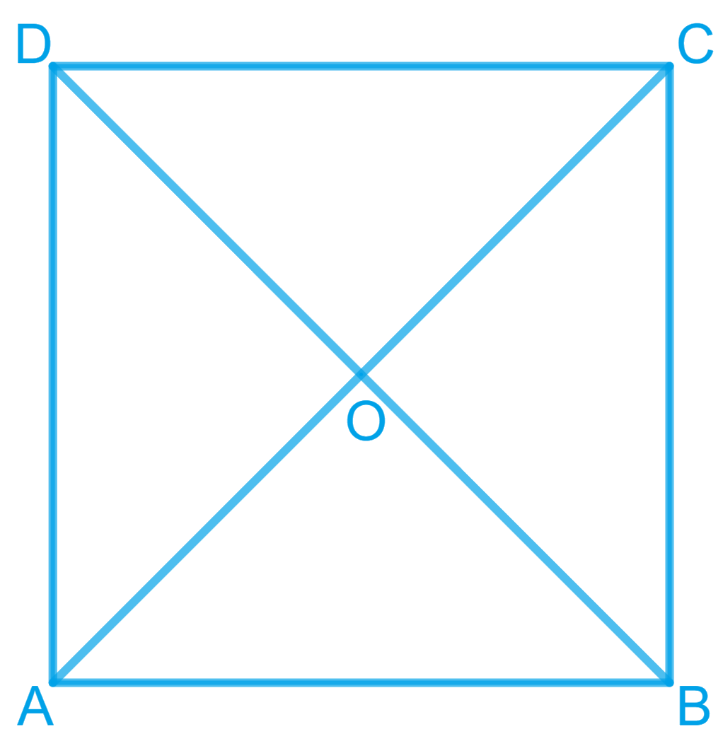Let $$ABCD$$ be a square. Let the diagonals $$AC$$ and $$BD$$ intersect each other at a point $$O$$. To prove that the diagonals of a square are equal and bisect each other at right angles, we have to prove,

\begin{align}&\mathrm{AC}=\mathrm{BD}, \mathrm{OA}=\mathrm{OC}, \mathrm{OB}=\mathrm{OD},\end{align} and \begin{align} \angle \mathrm{AOB}=90^{\circ} \end{align}

In $$\triangle \mathrm{ABC} \text { and } \Delta \mathrm{DCB}$$

\begin{align} AB&={DC}\left[\begin{array}{}\text {Sides of a square are}\\ \text{equal to each other}\end{array}\right]\\{\angle {ABC}}&\!=\!\angle {DCB} \left[\!\begin{array}{}\text{All interior angles }\\ \text{ are of }90^\circ \end{array}\!\right]\\ {{BC}}&={{CB} \quad \text{(Common side) }} \\ ∴ \Delta {ABC} &\cong \Delta {DCB} \left[\begin{array}{}\text{By SAS }\\ \text{congruency}\end{array}\right] \\ {∴ \; {AC}}&={{DB} \quad\text{(By CPCT )}}\end{align}

Hence, the diagonals of a square are equal in length.

In $$\Delta {{AOB \;\rm and }}\;\Delta {{COD ,}}$$

\begin{align}\angle {{AOB}} &= \angle {{COD}}\\\text{(Vertically }&\text {opposite angles)}\\\\\angle {{ABO}} &= \angle {{CDO}}\\\text{(Alternate}&\text { interior angles)}\\\\{{AB}} &= {{CD}}\\\text{( Sides of a square}&\text { are always equal)}\\\\\therefore \Delta {{AOB}}&\cong \Delta {{COD }}\\ \text{(By AAS}&\text { congruence rule)}\\\\ \therefore {{AO}} &= {{CO \;\rm and \;OB}} = {{OD}}\\ &\text{(By CPCT)}\end{align}

Hence, the diagonals of a square bisect each other.

In \begin{align} \Delta {AOB} \end{align} and \begin{align} \Delta {COB}, \end{align}

As we had proved that diagonals bisect each other, therefore,

\begin{align} {{AO}}&={{CO}} \\ {{AB}}&={CB}\\ \text{( Sides of a}&\text { square are equal )} \\ \\{{BO}}&={{BO} \,\quad \text{( Common )}}\\{∴\Delta {AOB} }&\cong \Delta {COB}\\ \text{(By SSS}&\text { congruency )} \\\\ \therefore {\angle {AOB}}&={\angle {COB}\,\quad \text{(By CPCT)}}\end{align}

However,

\begin{align}\angle {AOB}+\angle {COB}&=180^{\circ} \\2 \angle {AOB} &=180^{\circ} \\ \angle {AOB}&=90^{\circ}\end{align}

Hence, the diagonals of a square bisect each other at right angles.

## Chapter 8 Ex.8.1 Question 5

Show that if the diagonals of a quadrilateral are equal and bisect each other at right angles, then it is a square.

### Solution

What is known?

The diagonals of a quadrilateral are equal and bisect each other at right angle.

What is unknown?

How we can show that it is a square.

Reasoning:

We have to show that given quadrilateral is a parallelogram in which all sides are equal and one of its interior angles is .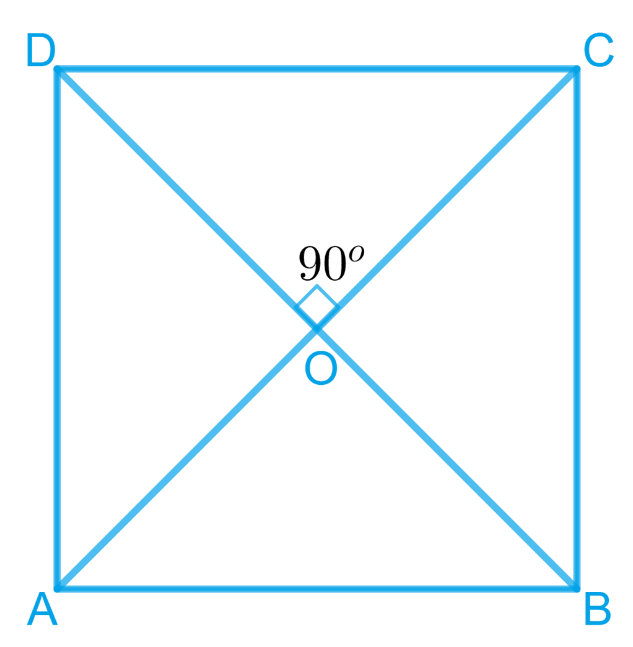Steps:

Let us consider a quadrilateral $$ABCD$$ in which the diagonals $$AC$$ and $$BD$$ intersect each other at $$O$$. It is given that the diagonals of $$ABCD$$ are equal and bisect each other at right angles.

Therefore,

$${\rm{AC }} = {\rm{ BD}},{\rm{ OA }} = {\rm{ OC}},{\rm{ OB }} = {\rm{ OD}},$$ and

$$\angle \,{\rm{AOB }} \!=\!\angle {\rm{BOC }} \!=\! \angle {\rm{COD }} \!=\! \angle {\rm{AOD }} \!=\! {\rm{ 9}}{0^0}$$

To prove $$ABCD$$ is a square

We have to prove that $$ABCD$$ is a parallelogram,in which

$$AB = BC = CD = AD$$, and one of its interior angles is $$90^{0}$$ .

In $${ \Delta{AOD} }{ {\: \rm and } \:\Delta {COD}}$$

\begin{align}{{AO}}&={CO}\\ \text{(Diagonals }& \text { bisect each other)} \\\\ \angle {AOD}&=\angle {COD}\\text{ Given that} &\text{ each is } 90^{\circ}) \\\\ {{OD}}&={{OD}\quad( \text{ Common })}\\ \therefore \Delta {AOD} &\cong \Delta {COD}\\ \text{(SAS}& \text { congruence rule)} \\\\ \therefore {AD}&={{DC}}\quad\dots(3)\end{align} $\rm {And,}\angle \mathrm{OAB}=\angle \mathrm{OCD}(\mathrm{By} \,\text {CPCT})$ However, these are alternate interior angles for line \(AB and $$CD$$ and alternate interior angles are equal to each other only when the two lines are parallel.

$$AB \;|| \;CD$$... (2)

From Equations (1) and (2), we obtain $$ABCD$$ is a parallelogram.

In $$\Delta AOD$$ and $$\Delta {COD}$$

\begin{align} {{AO}}&={CO}\\ \text{(Diagonals }& \text { bisect each other)} \\ \\\ {\angle {AOD}}&=\angle {COD}\\text{ Given that} &\text{ each is } 90^{\circ})\\\\ {{OD}}&={{OD}\,\,( \text{ Common })}\\ \therefore \Delta {AOD} &\cong \Delta {COD}\\ \text{(SAS}& \text { congruence rule)} \\\\ \therefore {AD}&={{DC}}\qquad\dots(3)\end{align} However, \(AD = BC and $$AB = CD$$

(Opposite sides of parallelogram $$ABCD$$)

$$AB = BC = CD = DA$$

Therefore, all the sides of quadrilateral $$ABCD$$ are equal to each other.

In $$\Delta {ADC}$$ and $$\Delta {BCD} ,$$

\begin{align}\\ {{AD}}&={{BC}\; ( \text{ Already proved })} \\ {{AC}}&={{BD} \;\text{ (Given) }} \\ {{DC}}&={{CD}\; ( \text{ Common })}\\{\therefore \Delta {ADC} }&\cong \Delta {BCD}\\ \text{(SAS}& \text { congruence rule)} \\\\ {\therefore\angle {ADC}}&={\angle {BCD}\;(\text{By} \,\text{CPCT})}\end{align}

However,

\begin{align} \angle {ADC}\!+\angle {BCD}&=180^{\circ}\\ \text{ (Co-interior }& \text {angles) } \\\\ {\qquad \angle {ADC}+\angle {ADC}}&={180^{\circ}}\\{2 \angle {ADC}}&={180^{\circ}} \\ {\therefore \angle {ADC}}&={90^{\circ}}\end{align}

One of the interior angles of quadrilateral $$ABCD$$ is a right angle.

Thus, we have obtained that $$ABCD$$ is a parallelogram, $$AB = BC = CD = AD$$ and one of its interior angles is $$90^{\circ}$$ .

Therefore, $$ABCD$$ is a square.

## Chapter 8 Ex.8.1 Question 6

Diagonal $$AC$$ of a parallelogram $$ABCD$$ bisects $$\angle A$$ (see the given figure). Show that

(i) It bisects $$\angle C$$ also,

(ii) $$ABCD$$ is a rhombus.

### Solution

What is known?

The diagonal AC of a parallelogram $$ABCD$$ bisects $$∠A.$$

What is unknown?

How we can show that (i) It bisects $$∠C$$ also, (ii) ABCD is a rhombus.

Reasoning:

We can use alternate interior angles property to show diagonal $$AC$$ bisects angle $$C$$ also, by showing all sides equal it can be said rhombus.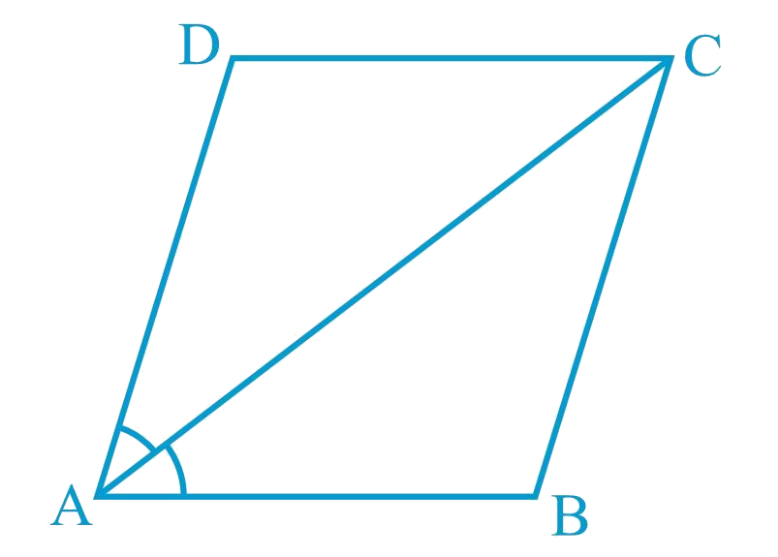Steps:

(i) $$ABCD$$ is a parallelogram.

\begin{align} &\angle \mathrm{DAC}=\angle {BCA}\\&\text{(Alternate interior angles are equal)} \ldots(1)\end{align}

\begin{align}\text{And,} &\angle \mathrm{BAC}=\angle {DCA}\\&\text{(Alternate interior angles are equal)} \ldots(2)\end{align}

However, it is given that $$AC$$ bisects $$\angle A$$.

$$\angle \mathrm{DAC}=\angle \mathrm{BAC} \ldots(3)$$

From Equations ($$1$$),($$2$$), and ($$3$$), we obtain

\begin{align} {\angle D A C}&\!=\!\!{\angle B C A\!=\!\!\angle B A C\!=\!\!\angle D C A \ldots\!(\!4\!)} \\ {\angle D C A}&\!=\!\!{\angle B C A}\end{align}

Hence, $$AC$$ bisects $$\angle C$$.

(ii) From Equation ($$4$$), we obtain

$$\angle {DAC}=\angle {DCA}$$

$${{DA}}={DC}$$(Side opposite to equal angles are equal)

However, $$DA = BC$$ and $$AB = CD$$

(Opposite sides of a parallelogram)

$$AB = BC = CD = DA$$

Hence, $$ABCD$$ is a rhombus.

## Chapter 8 Ex.8.1 Question 7

$$ABCD$$ is a rhombus. Show that diagonal $$AC$$ bisects $$\angle A$$ as well as $$\angle C$$ and diagonal $$BD$$ bisects $$\angle B$$ as well as $$\angle D$$.

### Solution

What is known?

ABCD is a rhombus.

What is unknown?

How we can show that diagonal $$AC$$ bisects $$∠A$$ as well as $$∠C$$ and diagonal BD bisects $$∠B$$ as well as $$∠D.$$

Reasoning:

We can use alternate interior angles property to show diagonal $$AC$$ bisects angles $$A$$ and $$C$$, similarly diagonal $$BD$$ bisects angles B and D.

Steps: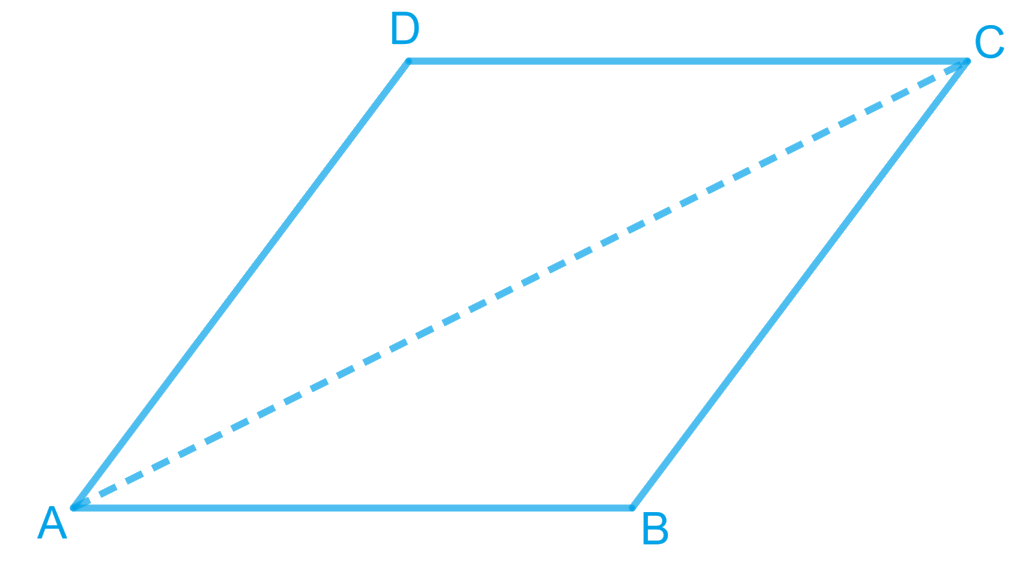Let us join $$AC$$.

In $$\Delta$$$$ABC$$,

\begin{align}&BC=AB\\&\left( \begin{array} & \text{Sides of a rhombus are} \\ \text{ equal to each other} \\ \end{array} \right)\\\\&\angle1=\angle2\\&\left( \begin{array} & \text{Angles opposite to equal sides } \\ \text{ of a triangle are equal} \\ \end{array} \right)\end{align}

However, $$\angle 1 = \angle 3$$ (Alternate interior angles for parallel lines $$AB$$ and $$CD$$)

$$\angle 2 = \angle 3$$

Therefore, $$AC$$ bisects $$\angle C$$.

Also, $$\angle 2 = \angle 4$$ (Alternate interior angles for || lines $$BC$$ and $$DA$$) $$\angle 1 = \angle 4$$

Therefore, $$AC$$ bisects $$\angle A$$.

Similarly, it can be proved that $$BD$$ bisects $$\angle B$$ and $$\angle D$$ as well.

## Chapter 8 Ex.8.1 Question 8

$$ABCD$$ is a rectangle in which diagonal $$AC$$ bisects $$\angle A$$as well as $$\angle C$$. Show that:

(i) $$ABCD$$ is a square

(ii) Diagonal $$BD$$ bisects $$\angle B$$ as well as $$\angle D$$.

### Solution

What is known?

$$ABCD$$ is a rectangle in which diagonal $$AC$$ bisects $$∠A$$ as well as $$∠C.$$

What is unknown?

How we can show that

(i) $$ABCD$$ is a square

(ii) Diagonal $$BD$$ bisects $$∠B$$ as well as $$∠D.$$

Reasoning:

We can use angle bisector property and isosceles triangle property to show given rectangle as square and alternate interior angles property to show BD bisects angles B and D.

Steps: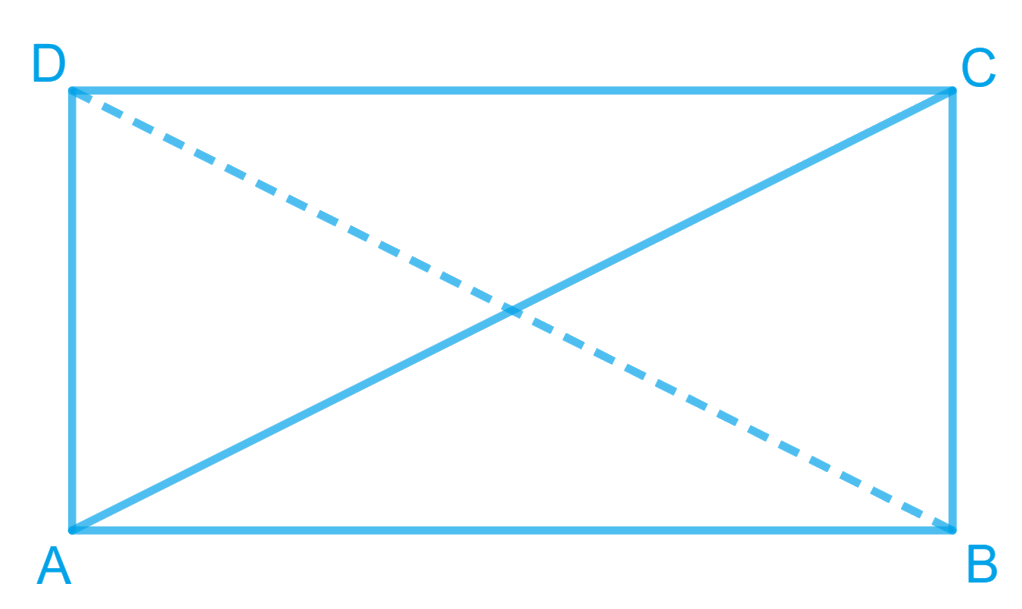(i) It is given that $$ABCD$$ is a rectangle.

\begin{align}\angle {\rm{A}} &= \angle {\rm{C}}\\\Rightarrow \frac{1}{2}\angle {\rm{A}} &= \frac{1}{2}\angle {\rm{C}}\\\Rightarrow \angle \rm DAC &= \frac{1}{2}\angle {\rm{DCA }} \{\text{AC bisects }}&\angle {\rm{A \,and }}\angle\,\, {\rm{C}})\end{align} \(CD = DA (Sides opposite to equal angles are also equal)

However, $$DA = BC$$ and $$AB = CD$$

(Opposite sides of a rectangle are equal)

$$AB = BC = CD = DA$$

$$ABCD$$ is a rectangle and all the sides are equal.

Hence, $$ABCD$$ is a square.

(ii) Let us join $$BD$$

In $$\Delta {BCD}$$

$$BC=CD$$ (Sides of a square are equal to each other)

$$\angle CDB=\angle CBD$$(Angles opposite to equal sides are equal)

However, $$\angle CDB = \angle ABD$$ (Alternate interior angles for $$AB \;||\; CD$$)

$\angle CDB = \angle ABD$

$$BD$$ bisects $$\angle B$$

Also, $$\angle CDB = \angle ABD$$ (Alternate interior angles for $$BC \;||\; AD$$) $$\angle CDB = \angle ABD$$

$$BD$$ bisects $$\angle D$$ and $$\angle B$$.

## Chapter 8 Ex.8.1 Question 9

In parallelogram $$ABCD$$, two points $$P$$ and $$Q$$ are taken on diagonal $$BD$$ such that

$$DP = BQ$$ (see the given figure). Show that: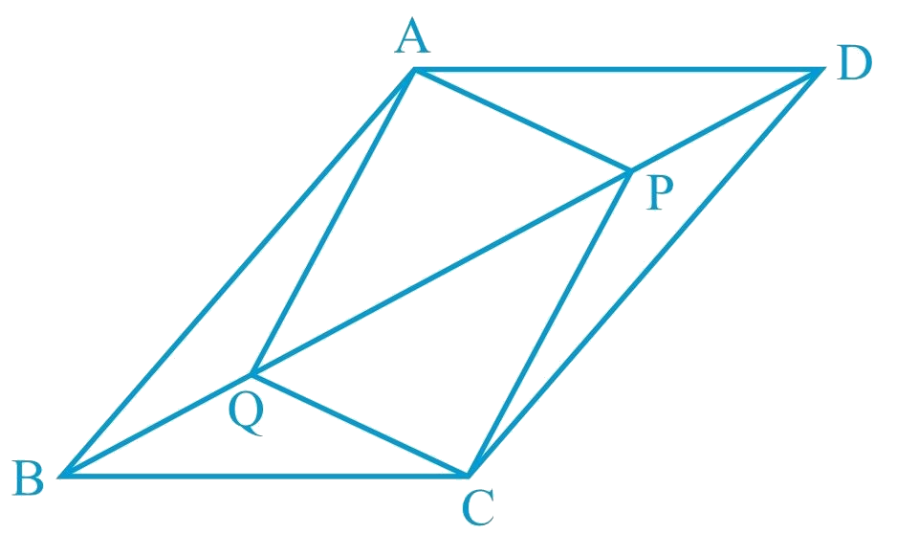\begin{align}&{\text { (i) } \triangle \mathrm{APD} \cong \Delta \mathrm{CQB}} \\ &{\text { (ii) } \mathrm{AP}=\mathrm{CQ}} \\ &{\text { (iii) } \triangle \mathrm{AQB} \cong \Delta \mathrm{CPD}} \\ &{\text { (iv) } \mathrm{AQ}=\mathrm{CP}} \\ &{\text { (v) APCQ is a parallelogram }}\end{align}

### Solution

What is known?

$$ABCD$$ is a parallelogram and $$DP = BQ$$

What is unknown?

How we can show that

\begin{align} &\left(\text{i} \right)\text{ }\ \ \ \Delta APD\cong \Delta CQB \\ &\left( \text{ii} \right)\text{ }\ \ AP=CQ \\ &\left( \text{iii} \right)\text{ }\ \Delta AQB\cong \Delta CPD \\ &\left(\text{ iv} \right)\text{ }\ AQ=CP \\ &\left( \text{v} \right)\text{ }\ APCQ\text{ }is\text{ }a\text{ parallelogram} \end{align}

Reasoning:

We can use alternate interior angles and parallelogram property for triangles congruence criterion to show triangles congruent then we can say corresponding parts of congruent triangles will be equal.

Steps:

(i) $$In{\text{ }}\Delta APD\;{\text{and}}{\text{ }}\Delta CQB,$$

\begin{align} \angle \mathrm{ADP} &\!=\!\angle \mathrm{CBQ}\!\begin{bmatrix}\text {Alternate interior}\\ \text{angles for BC } \| \mathrm{AD}\!\end{bmatrix} \\ \mathrm{AD}&\!=\!\mathrm{CB}\begin{bmatrix}\text {Opposite sides of} \\\text{parallelogram } \mathrm{ABCD}\end{bmatrix} \\ \mathrm{DP} &=\mathrm{BQ}\;(\text {Given}) \\ \therefore\triangle \mathrm{APD} &\cong \Delta \mathrm{CQB}\;\begin{bmatrix}\text { Using SAS }\\\text{congruence rule }\end{bmatrix} \end{align}

(ii) As we had observed that

$$\Delta APD \cong \Delta CQB$$

\begin{align} \therefore\, \mathrm{AP}=\mathrm{CQ}(\mathrm{CPCT})\end{align}

(iii) In $$\Delta AQB\;{\text{and}}{\text{ }}\Delta CPD,$$

\begin{align}{\angle \mathrm{ABQ}}&\!=\!\angle \mathrm{CDP}\!\begin{bmatrix}\! \text {Alternate interior}\\\text{ angles for } \mathrm{AB} \| \mathrm{CD} \!\end{bmatrix} \\ {\mathrm{AB}}&\!=\!\mathrm{CD}\begin{bmatrix}\text {Opposite sides of} \\\text{parallelogram } \mathrm{ABCD}\end{bmatrix} \\ {\mathrm{BQ}}&={\mathrm{DP}\;(\text {Given})} \\ {\therefore \Delta \mathrm{AQB} }&\cong \triangle \mathrm{CPD}\;\begin{bmatrix}\text { Using SAS }\\\text{congruence rule }\end{bmatrix} \end{align}

(iv) As we had observed that

$$\Delta AQB \cong \Delta CPD,$$

\begin{align}\therefore \mathrm{AQ}=\mathrm{CP}(\mathrm{CPCT})\end{align}

(v) From the result obtained in (ii) and (iv),

\begin{align} \text { AQ }&=\mathrm{CP} \text { and } \\ {\mathrm{AP}}&={\mathrm{CQ}}\end{align}

Since opposite sides in quadrilateral $$APCQ$$ are equal to each other, $$APCQ$$ is a parallelogram.

## Chapter 8 Ex.8.1 Question 10

$$ABCD$$ is a parallelogram and $$AP$$ and $$CQ$$ are perpendiculars from vertices $$A$$ and $$C$$ on

diagonal $$BD$$ (See the given figure). Show that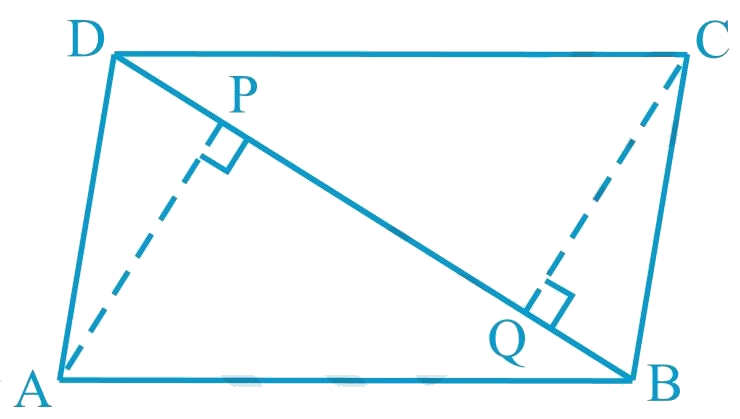(i) \begin{align} &{ \Delta \mathrm{APB} }{\cong \Delta \mathrm{CQD}} \end{align}

(ii) \begin{align} &{ \mathrm{AP}=\mathrm{CQ}}\end{align}

### Solution

What is known?

$$ABCD$$ is a parallelogram and $$AP\bot DB,\,CQ\bot DB$$

What is unknown?

How we can show that (i) $$\Delta APB\cong \Delta CQD$$

(ii) $$AP = CQ$$

Reasoning:

We can use alternate interior angles and parallelogram property for triangles congruence criterion to show triangles congruent then we can say corresponding parts of congruent triangles will be equal.

Steps:

(i) In \begin{align} \;&\Delta \mathrm{APB} \text { and } \Delta \mathrm{CQD} \end{align}

\begin{align}\angle \mathrm{APB}&=\angle \mathrm{CQD}\begin{bmatrix} \text {Each angle} \\ \text{measures } 90^{\circ} \end{bmatrix} \\ {AB}&\!=\!{CD} \begin{bmatrix}\text {Opposite sides of} \\\text{parallelogram } {ABCD}\end{bmatrix} \\ \angle \mathrm{ABP}&\!=\!\angle \mathrm{CDQ} \begin{bmatrix}\text {Alternate interior}\\ \text{angles for AB } \| \mathrm{CD}\!\end{bmatrix} \\ \therefore \Delta \mathrm{APB} &\cong \Delta \mathrm{CQD}\begin{bmatrix}\text { By AAS }\\\text{congruence rule }\end{bmatrix} \end{align}

(ii) By using the above result

\begin{align} \triangle \mathrm{APB} \cong \Delta \mathrm{CQD}, \end{align} we obtain \begin{align} \mathrm{AP}=\mathrm{CQ}\end{align} (By CPCT )

## Chapter 8 Ex.8.1 Question 11

In $$\Delta ABC \,\rm{and} \,\Delta DEF$$, $$AB \;|| \;DE$$,

$$BC = EF$$ and $$BC\: || \:EF$$. Vertices $$A$$, $$B$$ and $$C$$ are joined to vertices $$D$$, $$E$$ and $$F$$ respectively (see the given figure). Show that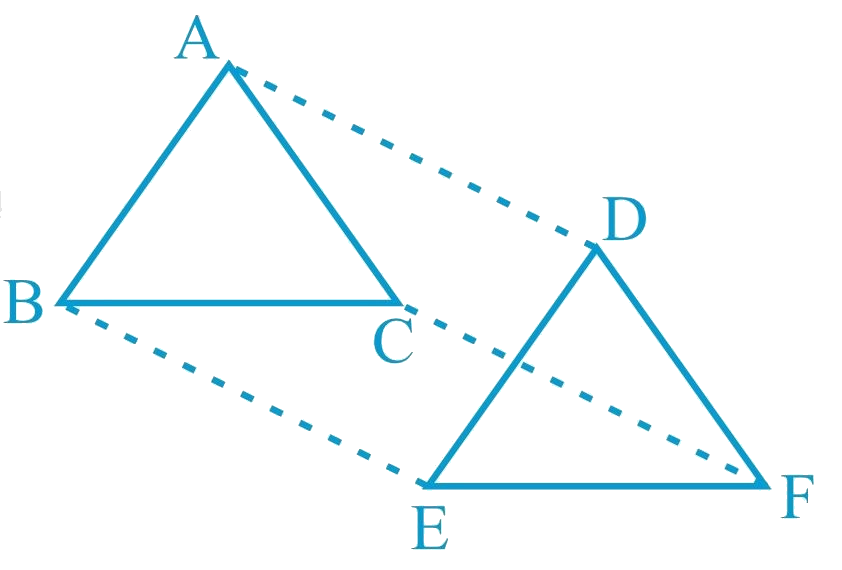(i) Quadrilateral $$ABED$$ is a parallelogram

(ii) Quadrilateral $$BEFC$$ is a parallelogram

(iii) \begin{align} &{ \mathrm{AD} \| \mathrm{CF} \text { and } \mathrm{AD}=\mathrm{CF}} \end{align}

(iv) Quadrilateral $$ACFD$$ is a parallelogram

(v) $$AC = DF$$

(vi) \begin{align} &{ \triangle \mathrm{ABC} \cong \triangle \mathrm{DEF} \text { . }}\end{align}

### Solution

What is known?

In $$\Delta ABC\text{ }and\text{ }\Delta DEF,$$

$$AB = DE, AB || DE, BC = EF$$ and $$BC || EF.$$

What is unknown?

How we can show that

(i) Quadrilateral $$ABED$$ is a parallelogram

(ii) Quadrilateral $$BEFC$$ is a parallelogram

(iii) $$AD || CF$$ and $$AD = CF$$

(iv) Quadrilateral $$ACFD$$ is a parallelogram

(v) $$AC = DF$$

(vi) $$\Delta ABC\cong \Delta DEF.$$

Reasoning:

We can use the fact that in a quadrilateral if one pair of opposite sides are parallel and equal to each other then it will be a parallelogram and converse is also true. Also by using suitable congruence criterion we can show triangles congruent then we can say corresponding parts of congruent triangles will be equal.

Steps:

(i) It is given that $$AB = DE$$ and $$AB \;|| \;DE$$.

If one pair of opposite sides of a quadrilateral are equal and parallel to each other, then it will be a parallelogram.

Therefore, quadrilateral $$ABED$$ is a

parallelogram.

(ii) Again, $$BC = EF$$ and $$BC\: || \:EF$$

Therefore, quadrilateral $$BCFE$$

is a parallelogram.

(iii) As we had observed that $$ABED$$ and $$BEFC$$ are parallelograms, therefore

$$AD = BE$$ and $$AD \;|| \;BE$$

(Opposite sides of a parallelogram are equal and parallel)

And, $$BE = CF$$ and $$BE\; || \;CF$$

(Opposite sides of a parallelogram are equal and parallel)

$$\therefore$$  $$AD = CF$$ and $$AD \;|| \;CF$$

(iv) As we had observed that one pair of opposite sides ($$AD$$ and $$CF$$) of quadrilateral $$ACFD$$ are equal and parallel to each other, therefore, it is a parallelogram.

(v) As $$ACFD$$ is a parallelogram, therefore, the pair of opposite sides will be equal and parallel to each other.

$$\therefore$$  $$AC\; || \;DF$$ and $$AC = DF$$

(vi) \begin{align} { \triangle \mathrm{ABC} \text { and } \triangle \mathrm{DEF} \text { , }} \end{align}

\begin{align} \mathrm{AB} &=\mathrm{DE}(\text {Given}) \\ \mathrm{BC} &=\mathrm{EF}(\text { Given}) \\ \mathrm{AC} &=\mathrm{DF}\\mathrm{ACFD} &\text { is a parallelogram) }\\ \therefore \triangle \mathrm{ABC} &\cong \Delta \mathrm{DEF}\\\text{(By SSS} &\text { congruence rule })\end{align} ## Chapter 8 Ex.8.1 Question 12 \(ABCD is a trapezium in which $$AB\; ||\; CD$$ and $$AD = BC$$ (see the given figure). Show that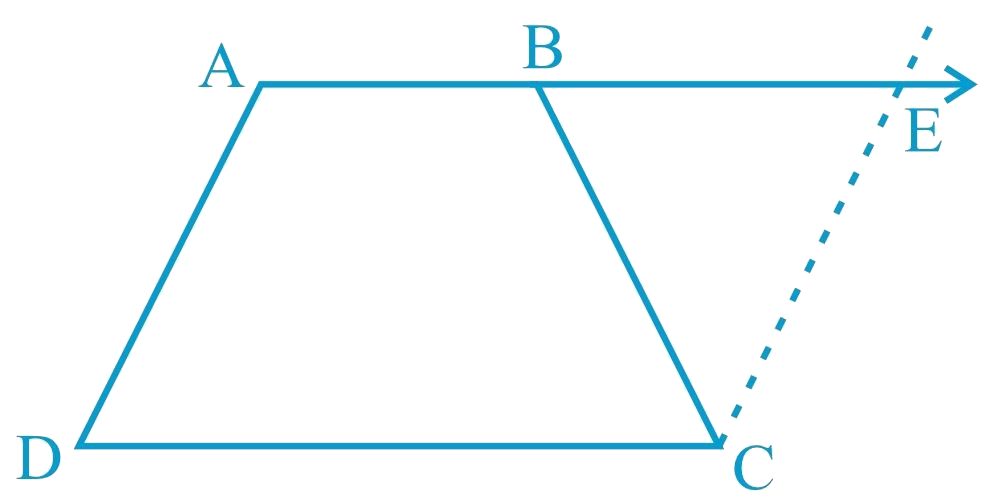(i) \begin{align} &{ \angle {A}=\angle {B}} \end{align}

(ii) \begin{align} & { \angle {C}=\angle {D}} \end{align}

(iii) \begin{align}&{ \triangle {ABC} \cong \triangle {BAD}} \end{align}

(iv) diagonal $$AC =$$ diagonal $$BD$$

[Hint: Extend $$AB$$ and draw a line through $$C$$ parallel to $$DA$$ intersecting $$AB$$ produced at $$E$$.]

### Solution

What is known?

$$ABCD$$ is a trapezium in which $$AB || CD$$ and $$AD = BC.$$

What is unknown?

How we can show that

(i) $$∠A = ∠B$$

(ii) $$∠C = ∠D$$

(iii) $$∆ABC ≅ ∆BAD$$

(iv) diagonal $$AC =$$ diagonal $$BD$$

Reasoning:

Consider parallel lines $$AD$$ and $$CE, AE$$ is the transversal line for them then sum of co-interior angles will be $$180$$ degree also angles $$CBE$$ and $$CBA$$ are linear pairs gives sum $$180$$, angles $$CEB$$ equals to $$CBE$$ because opposite to equal sides in triangle $$BCE$$. Using these observations angle A is equal to angle B. Then AB is parallel to CD will help to show angle C is equal to angle D. Also, by using suitable congruence criterion we can show triangles congruent then we can say corresponding parts of congruent triangles will be equal.

Steps:

Let us extend $$AB$$. Then, draw a line through $$C$$, which is parallel to $$AD$$, intersecting $$AB$$ at point $$E$$. It is clear that $$AECD$$ is a parallelogram.

(i) $$AD = CE$$ (Opposite sides of parallelogram $$AECD$$)

However, $${AD}={BC}(\text { Given })$$

Therefore, $${BC}={CE}$$

\begin{align}&\angle {{CEB}} = \angle {{CBE}}\\&\left( \begin{array}{l}{\text{Angle opposite to equal }}\\{\text{sides are also equal}}\end{array} \right)\end{align}

Consider parallel lines  and .  is the transversal line for them.

\begin{align}&\angle {{A}} + \angle {{CEB}} = {180^\circ }\\&\left( \begin{array}{l}{\text{Angles on the same }}\\{\text{side of transversal}}\end{array} \right)\end{align}

\begin{align}&\angle {{A}} + \angle {{CBE}} = {180^\circ }\\&\left( \begin{array}{l}{\text{Using the relation }}\\\angle {{CEB}} = \angle {{CBE}}\end{array} \right) \ldots (1)\end{align}

However, $$\angle B$$ + $$\angle CBE$$ = (Linear pair angles) ... (2)

From Equations (1) and (2), we obtain

$$\angle A$$ = $$\angle B$$

(ii)

\begin{align}&\angle A + \angle D = {180^\circ }\\&\left( \begin{array}{l}{\text{Angles on the same side}}\\{\text{ of the transversal}}\end{array} \right)\end{align}

Also,
\begin{align}&\angle C + \angle B = {180^\circ }\\&\left( \begin{array}{l}{\text{Angles on the same side }}\\{\text{of the transversal}}\end{array}\right)\end{align}

$\therefore \angle A + \angle D = \angle C + \angle B$

However,
\begin{align}&\angle A = \angle B\\&\left[ \begin{array}{l}{\text{Using the result }}\\{\text{obtained in (i) }}\end{array} \right]\\&\therefore \,\,\,\angle C = \angle D\end{align}

(iii) In \begin{align}\triangle {ABC} \text { and } \triangle {BAD}\end{align}

\begin{align}{{AB}} &= {{BA \text{(Common side) }}}\\{{BC}}& = AD\text {(Given) }\\\angle {{B}} &= \angle A {\text{(Proved before) }}\\\therefore \Delta {{ABC}} &\cong \Delta {{BAD }}\\&\left( {{\text{SAS congruence rule}}} \right)\end{align}

(iv) We had observed that,

\begin{align} \Delta {ABC}& \cong \Delta {BAD} \\ \;\;\;\;\therefore {AC}&= {{BD}({By} \;{CPCT})}\end{align}

Quadrilaterals | NCERT Solutions
Instant doubt clearing with Cuemath Advanced Math Program